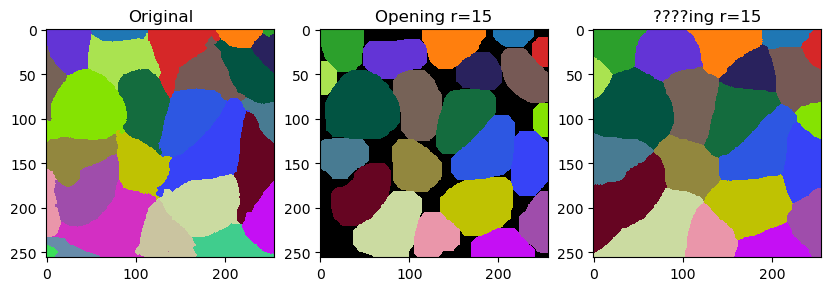# Smoothing labels#

In this notebook we will demonstrate how to fine-tune outlines of labels by smoothing them. The operation is related to erosion and dilation of labels. It is however not exactly what opening does. It differs by not leaving gaps behind but filling them with the closes labels.

import numpy as np
import pyclesperanto_prototype as cle
import matplotlib.pyplot as plt


A potential use-case is fine-tuning cell segmentation results. Thus, we take a look at a segmentation of cells based on membranes.

membranes = cle.imread("../../data/membranes.tif")
membranes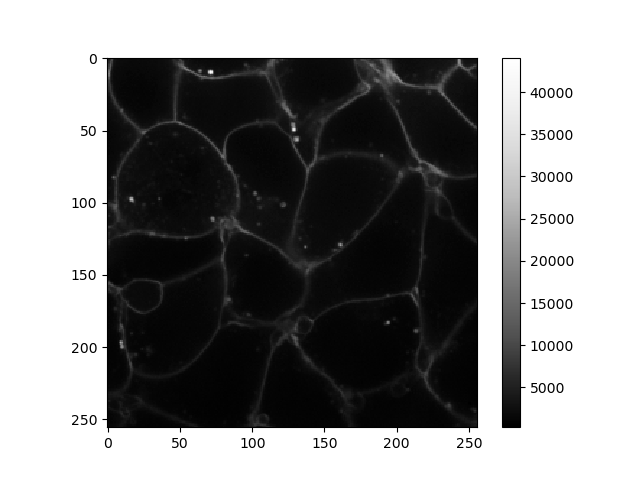cle._ image
 shape (256, 256) dtype float32 size 256.0 kB min 277.0 max 44092.0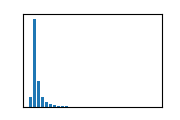labels = cle.imread("../../data/membranes_labeled.tif").astype(np.uint32)
labels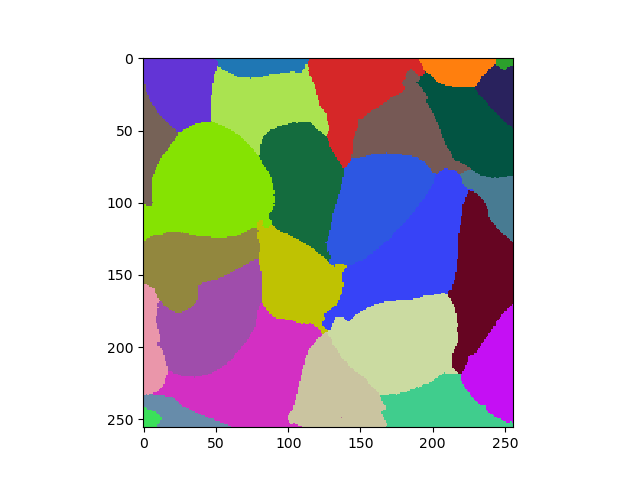cle._ image
 shape (256, 256) dtype uint32 size 256.0 kB min 1.0 max 28.0

The smooth_labels function allows to straighten the outlines of the labels.

cle.smooth_labels(labels, radius=5)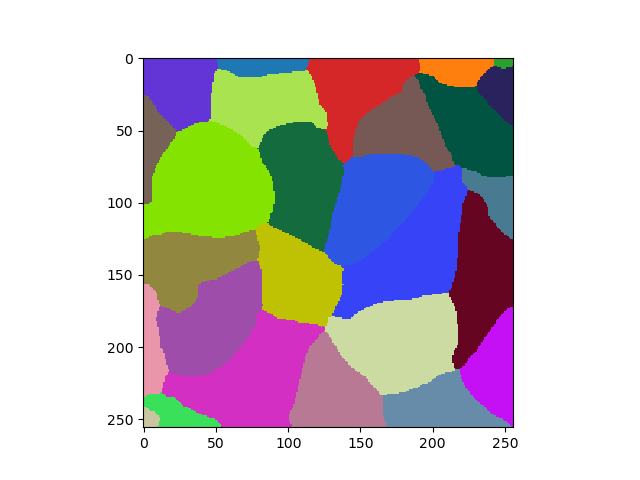cle._ image
 shape (256, 256) dtype uint32 size 256.0 kB min 1.0 max 27.0

The following code block is a modified version of the code that was used to generate the video in this tweet where we discussed the name of the filter.

# for r in range(20):
for r in range(0, 20, 5):

fig, ax = plt.subplots(1,3, figsize=(10,10))

cle.imshow(labels, labels=True, plot=ax)

ax.set_title("Original")
ax.set_title("Opening r=" + str(r))
ax.set_title("????ing r=" + str(r))
# plt.savefig("temp/" + str(r).zfill(2) + ".tif", dpi=300)
plt.show()

radius = 0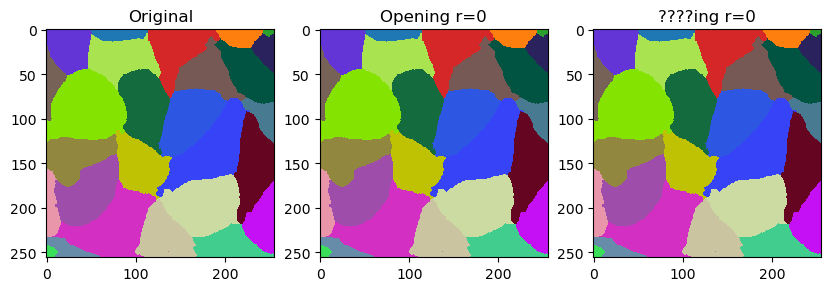radius = 5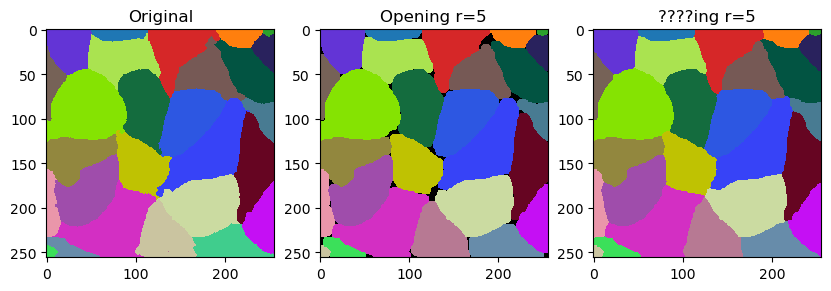radius = 10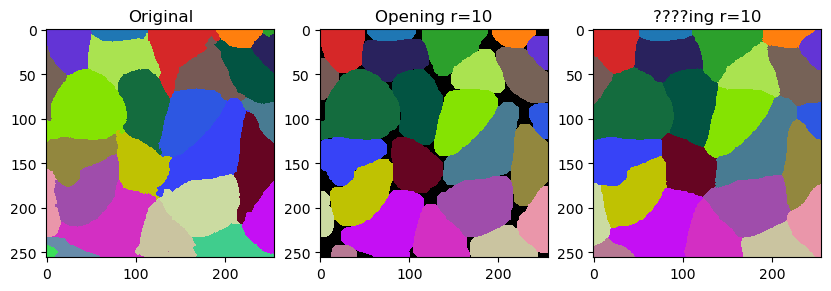radius = 15